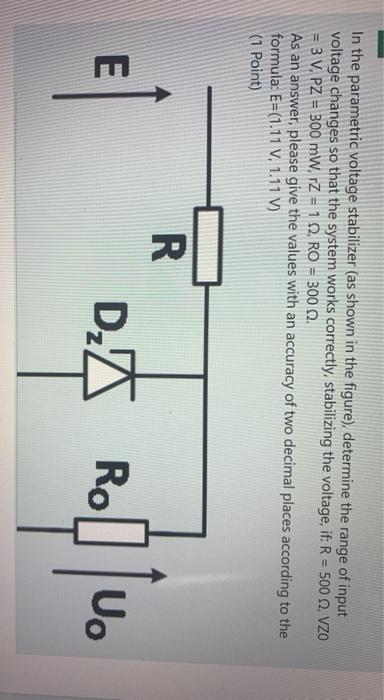# Question Solved1 AnswerIn the parametric voltage stabilizer (as shown in the figure), determine the range of input voltage changes so that the system works correctly, stabilizing the voltage, if. R = 500 2 VZO = 3 V, PZ = 300 mW, Z = 1 12, RO = 3000 As an answer, please give the values with an accuracy of two decimal places according to the formula: E=(1.11 V, 1.11 V) (1 Point) E 티 R DX Rol U.E5WUWG The Asker · Electrical EngineeringTranscribed Image Text: In the parametric voltage stabilizer (as shown in the figure), determine the range of input voltage changes so that the system works correctly, stabilizing the voltage, if. R = 500 2 VZO = 3 V, PZ = 300 mW, Z = 1 12, RO = 3000 As an answer, please give the values with an accuracy of two decimal places according to the formula: E=(1.11 V, 1.11 V) (1 Point) E 티 R DX Rol U.
More
Transcribed Image Text: In the parametric voltage stabilizer (as shown in the figure), determine the range of input voltage changes so that the system works correctly, stabilizing the voltage, if. R = 500 2 VZO = 3 V, PZ = 300 mW, Z = 1 12, RO = 3000 As an answer, please give the values with an accuracy of two decimal places according to the formula: E=(1.11 V, 1.11 V) (1 Point) E 티 R DX Rol U.